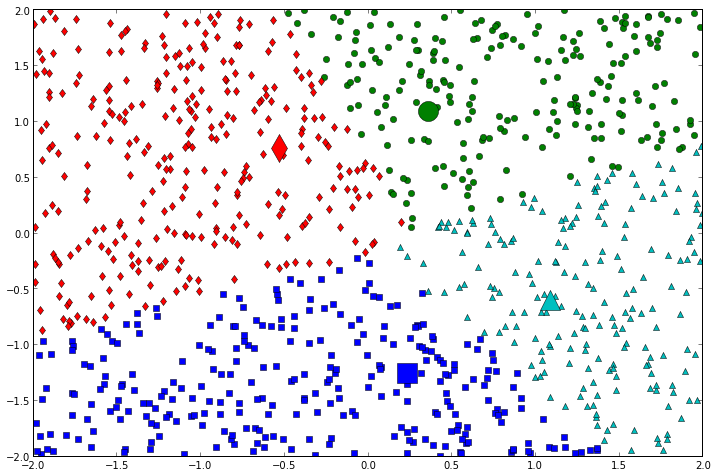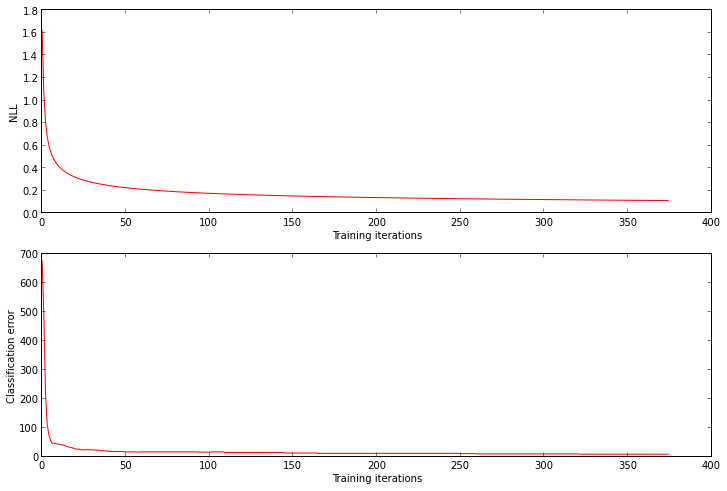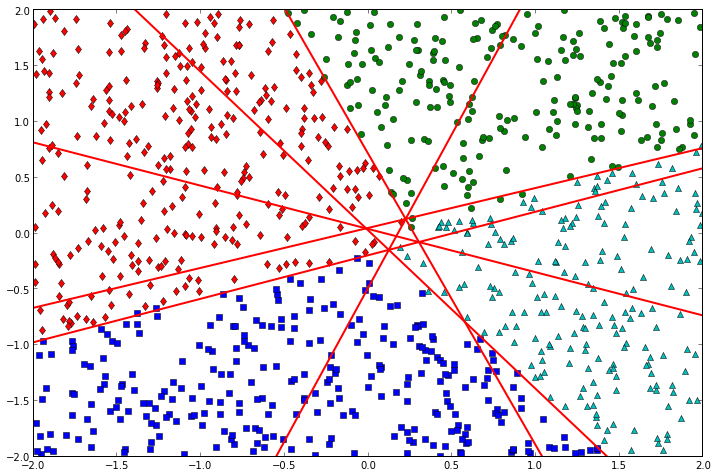# Autotelic Computing

## Saturday, October 12, 2013

### Softmax Regression 101

As this seems to be becoming a series of related posts, see also:
1. Linear Regression 101
2. Logistic Regression 101
3. Softmax Regression 101
4. Neural Network 101

## A Cloud of Points¶

If logistic regression allows to solve binary classification problems, softmax regression, our third example of a generalized linear model, allows to solve multiclass ones. As before, we build an artificial dataset, this time by first picking $k$ random points, each corresponding to a class. Next, we sample points uniformly, assigning each of them to the class to which it is closest to the center.
In :
def dataset(n, k):
xy = random.uniform(low=-2, high=2, size=(n, 2))
centers = random.uniform(low=-2, high=2, size=(k, 2))
classes = []
for p in xy:
classes.append(argmin([linalg.norm(p - c) for c in centers]))
return column_stack([xy, classes]), centers

random.seed(10)
k = 4
points, centers = dataset(1000, k)
colors =  'gbrcmykrcm'
markers = 'osd^v1234x'
for i in range(k):
class_i = points[where(points[:,2] == i)]
marker = colors[i % len(colors)] + markers[i % len(markers)]
plot(class_i[:,0], class_i[:,1], marker)
plot([centers[i,0]], [centers[i,1]], marker, ms=20)## Guessing an Initial Model¶

As before we will guess initial values for the $\theta$ parameters for our model, which will be, in the multiclass setting, a matrix of $k$ rows, each one having 3 columns, and thus corresponding to the parameters of a linear equation in the general form ($ax + by + c = 0$), as with logistic regression.
In :
initial_theta = random.sample(size=(k, 3))

This time however, we will postpone the question of making geometric sense of those parameters, as it is a bit more involved than with logistic regression (we'll come back to it in the end, when the model is trained).

## Training to Get Better¶

As softmax regression is still (as all generalized linear models are) a probabilistic method, this time we will use the softmax function to squash the activation of class $i$ into a probability value
$h(x, i) = \frac{e^{x_i}}{\sum_{j}^{k} e^{x_j}}$
The error function for softmax regression is a generalization of the NLL we've been using for logistic regression
$NLL = - \sum_{i}^{n} \log \prod_{j}^{k} h_{\theta_j}(\vec{x^{(i)}})^{1\{c^{(i)}=j\}}$
The derivative of the NLL, with respect to $\theta$ again follows the familiar form, with the difference that it is now two-dimensional
$\frac{\partial NLL}{\partial \theta_{ij}} = (c - h_{\theta_{i}}(\vec{x})) \cdot \vec{x_{i,j}}$
In :
def softmax(x, theta):
n = len(x)
k = len(theta)
sum_exps = sum(exp(asarray([dot(x, theta[i]) for i in range(k)])), axis=0)
assert sum_exps.shape == (n,)
# k x n, where each col should sum to 1
return asarray([exp(dot(x, theta[i])) / sum_exps for i in range(k)])

def train(points, initial_theta, alpha=0.001, stop=1e-4):
x, y, c = points[:,0], points[:,1], points[:,2]
n = len(points)
k = len(initial_theta)
bxy = column_stack([ones(n), x, y]) # first component is intercept (i.e. all 1s)
thetas = [initial_theta]
nll_errors = []
classif_errors = []
while True:
theta = thetas[-1][:] # copy
h = softmax(bxy, theta)
nll_errors.append(-sum(log(h.T[arange(n), c.astype(int)])) / n)
classif_errors.append(sum((argmax(h, axis=0) != c).astype(int)))
if len(nll_errors) > 1 and nll_errors[-2] - nll_errors[-1] < stop: break
for i in range(k):
ci = (c == i).astype(int)
theta[i] -= alpha * sum(h[i] - ci) # intercept component
theta[i] -= alpha * sum((h[i] - ci) * x)
theta[i] -= alpha * sum((h[i] - ci) * y)
thetas.append(theta)
return thetas, nll_errors, classif_errors

thetas, nll_errors, classif_errors = train(points, initial_theta)

Let's verify that the NLL and the classification errors are getting minimized.
In :
_, axs = subplots(2, 1)
axs.set_xlabel('Training iterations')
axs.set_ylabel('NLL')
axs.plot(range(len(nll_errors)), nll_errors, 'r-')
axs.set_xlabel('Training iterations')
axs.set_ylabel('Classification error')
axs.plot(range(len(classif_errors)), classif_errors, 'r-')
axs.set_ylim(0);To visualize the decision lines, we note that even though we have $k$ instances of (3-component) $\theta_i$ parameters (which it would be thus tempting to use directly), they don't actually correspond to what we are looking for. Rather, we must consider that as each class must be distinguished from all the other classes, we must be looking for ${{k}\choose{2}}$ decision boundaries, each yielding a pairwise comparison (note that comparing class $i$ with class $j$ is the same as the opposite, hence the use of combinations). For each comparison of class $i$ with class $j$, the decision line parameters can be retrieved as $\theta_{ij} = \theta_i - \theta_j$.

Even though I invested a good amount of time trying to understand why this last aspect works and what it means, I haven't found a satisfying answer, at least in the form of a good geometric intuition. If anyone can provide additional insight, please jump in.
In :
def general_to_slope_intercept(theta):
slope = -theta / theta
intercept = -theta / theta
return slope, intercept

best_theta = thetas[-1]

for i in range(k):
class_i = points[where(points[:,2] == i)]
marker = colors[i % len(colors)] + markers[i % len(markers)]
plot(class_i[:,0], class_i[:,1], marker)
slope, intercept = general_to_slope_intercept(best_theta[i])

from itertools import combinations

for comb in combinations(range(k), 2):
theta = best_theta[comb] - best_theta[comb]
slope, intercept = general_to_slope_intercept(theta)
plot(linspace(-2, 2), slope * linspace(-2, 2) + intercept,
'r-', linewidth=2)
axis((-2, 2, -2, 2));